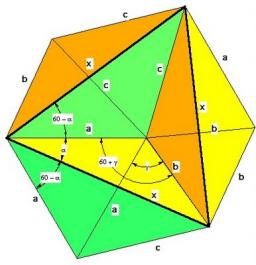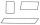# SAS triangle

The triangle has two sides long 7 and 19 and included angle 36°.

Calculate area of this triangle.

Correct result:

S =  34.4

#### Solution:

$S=\frac{1}{2}ah=\frac{1}{2}ab\mathrm{sin}\gamma =\frac{1}{2}13\cdot 9\cdot \mathrm{sin}3{6}^{\circ }=34.4$We would be pleased if you find an error in the word problem, spelling mistakes, or inaccuracies and send it to us. Thank you!Tips to related online calculators

#### You need to know the following knowledge to solve this word math problem:

We encourage you to watch this tutorial video on this math problem:

## Next similar math problems:

• Center traverseIt is true that the middle traverse bisects the triangle?
• Reference angleFind the reference angle of each angle:
• TrigonometryIs true equality? ?
• LiftThe largest angle at which the lift rises is 16°31'. Give climb angle in permille.
• Height 2Calculate the height of the equilateral triangle with side 38.
• SinesIn ▵ ABC, if sin(α)=0.5 and sin(β)=0.6 calculate sin(γ)
• CosineThe point (8, 6) is on the terminal side of angle θ. cos θ = ?
• Parallelogram +ľ| AB | = 76cm, | BC | = 44cm, angle BAD = 30 °. Find the area of the parallelogram.
• High wallI have a wall 2m high. I need a 15 degree angle (upward) to second wall 4 meters away. How high must the second wall?
• TreeHow tall is the tree that observed in the visual angle of 52°? If I stand 5 m from the tree and eyes are two meters above the ground.
• The cable carThe cable car has a length of 3,5 kilometers and an angle of climb of 30 degrees. What is the altitude difference between Upper and Lower Station?
• Equilateral triangleHow long should be the minimum radius of the circular plate to be cut equilateral triangle with side 19 cm from it?
• ReflectorCircular reflector throws light cone with a vertex angle 49° and is on 33 m height tower. The axis of the light beam has with the axis of the tower angle 30°. What is the maximum length of the illuminated horizontal plane?
• The cable carThe cable car is 2610 m long and rises at an angle of 35°. Calculate the height difference between the lower and upper station of the cable car.
• Cable car 2Cable car rises at an angle 41° and connects the upper and lower station with an altitude difference of 1175 m. How long is the track of cable car?
• The staircaseThe staircase has a total height of 3.6 m and forms an angle of 26° with the horizontal. Calculate the length of the whole staircase.
• RhomboidCalculate the circumference and area of the rhomboid with sides 20 and 14, with their angle 50°.